Next: Tri-state buffer based technique Up: Reducing Spurious Switching Activity Previous: Reducing Spurious Switching Activity

## Signal gating with filler values

If one part of a circuit is not doing anything useful in a given cycle, ideally its inputs should be frozen, i.e., remain unchanged. Various options exist for ensuring this. Transparent latches were proposed in . However, often the power savings obtained may not justify the large overhead introduced by multi-bit latches. Another option may be to set a input to tri-state when it is not active. Unfortunately, the tri-state gate output will usually drift to some mid-way voltage value if the gate is not refreshed in a short time. Such a mid-way value may cause large power consumption in downstream DPUs. Tri-state gating is justified only in very limited cases, which will be addressed later. In , it was mentioned that freezing the inputs to zero (AND gated) or one (OR gated) also reduces SSA. To make full use of data correlations inside the datapath, we propose to freeze the inputs to a fixed (hardwired) value. We call this value the filler value f. For the RTL circuit in Fig. 4(b), Fig. 9 gives the names of the variables whose values are propagated from the multiplier to register Reg2 after gating in one iteration of the CDFG, under four different scenarios. Z refers to tri-state (high-impedance), X implies that the value is not yet stable or visible at the RTL, y1' is the value of variable y1 in the previous iteration and `-' implies that the value remains unchanged. It is obvious that the SSA will be significantly suppressed by gating.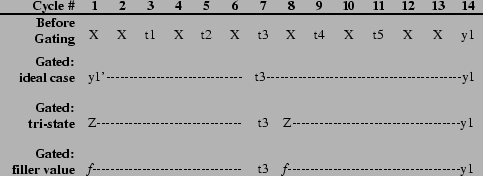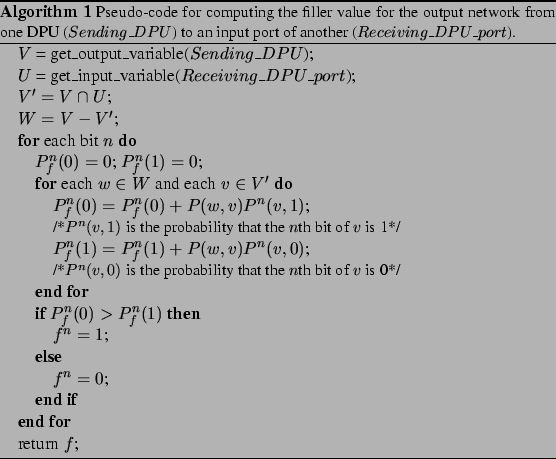Algorithm 1 contains the pseudo-code for computing the filler value to minimize interconnect switching activity. Suppose the set of variables sent by DPU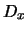(Sending_DPU) to all the DPUs in its output network is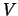. Let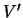denote the subset ofwhich contains all the variables used by an input port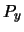of another DPU (Receiving_DPU_port). Variables inare called desired variables for. For the RTL circuit in Fig. 4(b),for the multiplier's output network is {t1,t2,t3,t4,y5,y1} and itsfor register Reg2 is {t3,y1}. For variables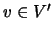and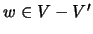, we obtain the probability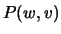that values ofand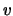will be output consecutively, irrespective of their order.can be computed by simulating the RTL circuit. Moreover, this simulation can also yield the probability for the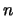th bit ofto be 1 and 0, respectively, whenis output right before or after any variable from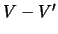. Let us denote these probabilities by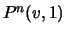and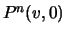, respectively. Note each value ofwill have a lifetime of consecutive cycles. That is,will hold the same value for these cycles. These consecutive cycles are counted as one single occurrence of the corresponding value when calculating the probabilities, because only transitions fromtowill consume dynamic power. Sinceis not used byand will be replaced by the filler value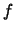, we need to decide whatshould be in order to minimize the switching activity. For theth bit of, say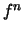, transitions take place when it is different from the values of the desired variables which are output right before or after it. The probabilities of transition whenis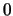and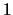,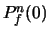and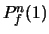, are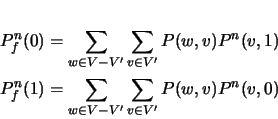If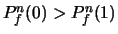,is set to 1, othervise 0. Thus, we can statistically minimize the bit transition activity in the output network from DPUto portby introducing the filler value. Since the switched capacitance is highly dependent on switching activity in the physically neighboring wires (due to coupling), we cannot say that the filler value thus chosen will yield minimal spurious switched capacitance in the wires. However, it has been found to reduce spurious switched capacitance significantly. When the data are totally random,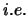,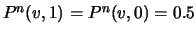, the optimal filler value can be either 1 or 0, which reduces to the method in . The overhead for setting a input to a fixed value is very low compared to the overhead for latches. One AND gate is enough for 0 and one OR gate for 1.Next: Tri-state buffer based technique Up: Reducing Spurious Switching Activity Previous: Reducing Spurious Switching Activity
Lin Zhong 2003-10-11